## Generalized Instant Insanity

The last problem in the “Games and Puzzles” section comes from a commercial puzzle (though one based on a much older puzzle).  It’s cool how these sorts of things pop up- a toy or game comes out and then people analyze it mathematically and find some neat features about it.

The problem: Generalized Instant Insanity.  This is problem GP15 in the appendix.

The description: Given a set Q of cubes, and a set C of colors we paint on the sides of each cube (and where |Q| = |C|) is there a way to arrange the cubes in a stack such that each color in C appears exactly once on each side?

Example: The Wikipedia page for the Instant Insanity game has a good description of the game and an example of the solution when Q = 4.

Reduction: Roberson and Munro reduce from “Exact Cover”.  I don’t think I’ve done this problem, but it’s basically X3C where the sizes of the sets can be any size.  (So it’s a trivial reduction from X3C).

They start by designing a graph representation of the puzzle, similar to the one shown on the Wikipedia page. Vertices correspond to colors, an edge connects two vertices if they are on opposite sides of the came cube, and the edges are labeled with the name of the cube.  The puzzle has a solution if and only if we can find two edge disjoint cycles that touch each vertex and edge label exactly once.  The two cycles correspond to the two sets of opposite faces we can see (since 2 sides of each cube are hidden by the stack).  Again, go look at the graphs in the Wikipedia article– they show this pretty nicely.

So, to perform the reduction, we’re given a set S {s1..sm} and a collection T of subsets of S, and need to make a graph (which corresponds to a puzzle).  Each element in S will become a vertex in the graph.  Each of these vertices will have a self-loop and be labeled ζi (So each vertex is a different color, and each self-loop is part of a different cube- so each cube has one color on opposite sides).

Each element si inside a set Th of T will also have a vertex: vi,h. This vertex has an edge with the next largest level sj within Th, wrapping around if we’re at the last element of Th. These edges are labeled ϒh,j.  We also have an edge from sjto vh,j if sj is in Th labeled with δh,j.  We also have 2 copies of self-loops from each vh,j to itself labeled ϒh,j.

They then add some extra edges to give the graph the properties:

• One of the self-loops from vh,i to itself has to be in one of the two cycle sets.
• If the edge from vh,i to sj (labeled ϒh,j) is in the other cycle set, then the edge from sj to vh,j (labeled δh,j) is also in that cycle set
• The ζi edge label has to be used, and so will have to be used on an si vertex the cycle that uses that vertex will also have to have the labels ϒh,j and δh,j for some h.
• The loops (labeled ζj and ϒh,j) are in one cycle set and the paths (labeled ϒh,j and δh,j) are in the other.

With these properties, then solving the puzzle means we have to find h1 through hm that correspond to sets Th in T, but also where there is a path ϒh,jh,j in the graph for each sj in Th_i.  We make sure no elements in S are repeated by making sure the puzzle only uses each sj vertex once.

Difficulty: 8.  I glossed over a lot of the construction, especially the definition of a “p-selector”, which is a subgraph that helps set up a lot of the properties above.  That definition is very hard for me to follow.

## Crossword Puzzle Construction

Closing in on the end of the section, this is a “private communication” problem that I think I figured out myself.

The problem: Crossword Puzzle Construction.  This is problem GP14 in the appendix.

The description: Given a set W of words (strings over some finite alphabet Σ), and an n x n matrix A, where each element is 0 (or “clear”) or 1 (or “filled”).  Can we fill the 0’s of A with the words in W?  Words can be horizontal or vertical, and can cross just like crossword puzzles do, but each maximally contiguous horizontal or vertical segment of the puzzle has to form a word.

Example: Here’s a small grid.  *’s are 1, dashes are 0:

 * – – – * – – – – – – – – – – * * – – * * * * – *

Suppose our words were: {SEW, BEGIN, EAGLE, S, OLD, SEA, EGGO, WILLS, BE, SEA, NED}.  (Notice the lone “S” is a word.  That’s different from what you’d see in a normal crossword puzzle)

We can fill the puzzle as follows:

 * S E W * B E G I N E A G L E * * O L D * * * S *

Notice that we can’t use the first two letters of “BEGIN” as our “BE”, because the word continues along.  That’s what the “maximally contiguous” part of the definition is saying.

Reduction: From X3C. We’re given a set X with 3q elements, and a collection C of 3-element subsets of X.  We’re going to build a 3q x q puzzle with no black squares. (We’ll get back to making this a square in a minute)  Each word in W will be a bitvectorof length 3q, with a 0 in each position that does not have an element, and a 1 in the positions that do.  So, if X was {1,2,3,4,5,6,7,8,9} the set {1,3,5} would be 101010000

We also add to A the 3q bitvectors that have exactly one 1 (and 0’s everywhere else). The goal is to find a subset of C across the “rows” of the puzzle, such that the “columns” of the puzzle form one of the bitvectors.  If we can form each of the bitvectors, we have found a solution to X3C.  If we have a solution to X3C, we can use the elements in C’ and place them in the rows of the 3q x q puzzle block to come up with a legal crossword puzzle.

We’re left with 2 additional problems:  The grid needs to be a square, instead of a 3q x q rectangle, and the legal crossword puzzle solution needs to use all of the words in W, not the the ones that give us a C’.  We can solve both by padding the grid with blank squares.  Spaced out through the blank spaces are 1 x 3q sections of empty space surrounded by black squares.  We can put any word in C-C’ in any of these sections, and that’s where we’ll put the words that are not used.

(This also means we’ll have to add 3x|(C’-C)| 1’s and (3q-3)|(C’-C)| 0’s to our word list for all of the 1-length words in those  columns.)  Then we add enough blank spaces around the grid to make it a square.

Difficulty: 5 if I’m right, mainly because of the extra work you have to do at the end.  The comments in G&J say that the problem is NP-Complete “even if all entries in A are 0”, which is usually a hint that the “actual” reduction used an empty square grid.  I wonder if that reduction doesn’t have my hacky stuff at the end.

## Square Tiling

The reference in G&J is to an “unpublished result” (by Garey and Johnson themselves, with Papadimitriou).  I think the solution I found is not the one they are referring to.

The problem: Square Tiling.  This is problem GP13 in the appendix.

The description: Given a set C of colors, and a set T of tiles, where each of the 4 sides of the tile are colors from C (listed as a 4-tuple of (top, right, bottom, left) colors), and an integer N.  Can we tile an NxN square of tiles using tiles from C?  We can use a tile more than once, the tiles can’t be rotated, and adjacent edges need to match colors.

Example: Here are some example tiles I drew:We can tile these into a 3×3 grid like this: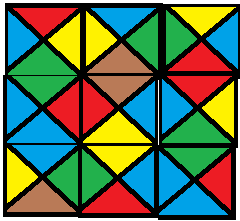Reduction: As I said above, the reference in G&J is to an “unpublished result” by Garey, Johnson, and Papadimitriou.  I did manage to find a “generic reduction” using Turing Machines in the Lewis and Papadimitriou Theory book.

The reduction is from the “N2” language in the book, which (I think) is “Can a Turing Machine M halt in t steps or less with its head on the tape in configration uσv, where u and v are strings in Σ*, and σ is the location of the head (and a symbol in the alphabet)?

The idea is that we’ll build a set of tiles whose colors are based on the number of steps the computation has done so far.  The colors are actually tuples.  So, we have several kinds of tiles:

• For each a in Σ*, and each k from 1 to t, a tile with color (a, k+1) on the top, and (a,k) on the bottom.  This simulates a move that stays in the same state and writes the same symbol.
• For each pair a,b in Σ*, and each state p,q in M (p can include the halt state, q can’t), a tile with the color (p,b,k+1) on the top and (q,a,k) on the bottom.  This simulates going from state p to state q and replacing an a with a b.
• We can transition from one of the above types of tiles to another using a sideways move.  (If the head moves left or right, we move to a tile to the left or right)
• There are special tiles for the start row and for when the machine halts.

We set our N (the size of the tiling grid we’re making) to t+2.  What we’ve built is a system that:

• Has to have the tiles corresponding to one of the start states on the bottom row
• Has in row i a tile corresponding to a configuration of the machine after i steps.  (A path of tiles from the bottom to row i show the computation needed to get to that state)
• Has at the top row a tile corresponding to a configuration after t+1 steps.  If there is a legal tiling, one of those tiles must contain the halt state.

..which they claim is the same as the N2 langauge.

The suggested reduction in the appendix is from Hamiltonian Path, and I spent some time thinking about how to make that work, but couldn’t do it.  Do you make tiles correspond to vertices? Do you make colors correspond to vertices?  How do you account for the fact that you can go in two dimensions?  How do you account for the fact that you don’t know the order in which you visit the tiles?  I think it might be similar to this way of thinking about configurations.

Difficulty: 9 because it’s such a non-standard way of doing things.  I bet the Hamiltonian Path reduction is a lot easier.

## Left-Right Hackenbush for Redwood Furniture

Here’s an interesting problem, but a hard one to explain.  Most of what I’m doing here comes from the very cool “Winning Ways for Your Mathematical Plays” book by Berlekamp, Conway, and Guy, which I hope at some point in the future to have the time to really dig deeply into.  But for now, I’ll just use it as a reference to this week’s problem.

The problem: Left-Right Hackenbush for Redwood Furniture.  This is problem GP12 in the appendix.

The description: Ok, here we go.  First, a Hackenbush problem consists of an undirected, connected graph.  The edges of the graph are marked as “Left” or “Right”(though the book has some very nice colored pictures, where the edges are labeled “Blue” and “Red”).  Some of the vertices are on the ground (In G&J’s definition, there is one ground vertex, but it’s equivalent to having several vertices that are all on the ground).

On Left’s turn, they remove a blue edge and then all edges that are not connected to the ground are removed.  On Right’s turn, they remove a red edge, and then all edges that are not connected to the ground are removed.  A player loses if there are no remaining edges of their color.

redwood furniture Hackenbush instance is one where:

• No red edges touch the ground
• Each blue edge (or “foot”) has one end on the ground and the other touches a unique red edge (the “leg”)

Here are some redwood furniture instances from the book: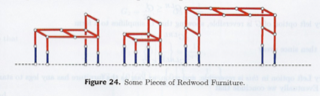The “value” of a Hackenbush position is the number of “spare” moves (with optimal play) one player has after the other player loses.  A value of 0 means that whoever’s turn it is will lose (on an empty board).  The definition can be extended to fractional values.  For example, a value of 1/2 for (say) Left means that if we made two copies of the game, we would end up with a situation with a value of 1 for Left.

So, the question is, for some Redwood Furniture graph, and some K, is the value <= 2-K?

Reduction:

I’m just going to sketch the process here since it takes several pages of the book (and depends on results and ideas from earlier in the book).

They show:

• The value of any redwood furniture graph is 2-N for some N.  In the degenerate case, the graph with just one Left edge has a value of 1. (=20)
• On Left’s turn, they will always remove a foot (by definition, that’s all they have).  On Right’s turn, they should make a move that does not disconnect the graph, if possible.
• A “Bed” is a redwood furniture graph where every red edge that is not a leg connects to a leg.  It has value 2-(m+1), where m is the largest number of moves that do not disconnect the bed.
• The value of the game depends on how many extra moves Red has to get down to just a bed.
• To find m, you need to know the size of the largest redwood tree (a tree is a graph that will be disconnected by the removal of any edge) that contains all of the legs.
• The edges of the bed (the red edges that are not legs) form a bipartite graph.  So finding m is equivalent to the set covering problem, where the elements of the set are the vertices, and the edges are the sets.

Here’s how I think the Set Covering reduction works.  Given a set covering instance: a set S of elements, and a collection C of subsets of S, we’ll build a bipartite graph.  One set of the bipartite graph will correspond to the elements of S (and will be on the legs of the furniture graph).  The other set will correspond to the elements in C (and will be the vertices in the bed that are not in the legs).  An edge will go from each “C vertex” to each “S vertex” in its set.  Now, the cover is the set of vertices from the bed that cover all of the legs.

The book says you want the “smallest redwood tree which contains all of the legs”, which I think is the same thing (smallest number of extra vertices), but I’m not 100% confident since the Hackenbush game involves removing edges, and we’re choosing vertices in the cover.

I’m a little sad that the book does such a great job describing the game, and the value function, and then glosses over the reduction part (and uses misleading terms like “Minimum Spanning Tree of a Bipartite Graph”, which is a polynomial problem).  The actual reduction in G&J is to a private communication, so that’s not much help.

Difficulty: Boy, I don’t know, I think it depends on where you start from.  If my Set Cover reduction is the right one, and all you ask a student to do is that, it’s probably a 4.  If you’re going to make them prove all of the things I glossed over about the value number, then it probably goes up to at least an 8.

## NxN Go

Similar to the last problem from the appendix in that we’re taking an actual game and extending it.

The problem: NxN Go.  This is problem GP10 in the appendix.

The description: Given an integer N, and a position (consisting of black piece locations, white piece locations, and black piece locations) on an NxN go board, and the name of the current player’s turn, does white have a forced win on the game?

Example: Instead of a true example, what I think is most relevant here is a little discussion of the rules and basic strategy of Go.  In Go, players take turns playing stones on a grid, with the goal of surrounding spaces on the board with pieces of your color:

(all pictures are taken from the tutorial at https://www.pandanet.co.jp/English/learning_go/learning_go_2.html):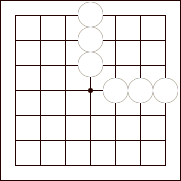In this case, white has surrounded 9 spaces (stones are placed at intersections, including the edges of the board).

If a stone of the other color can be surrounded on all 4 sides by a stone of your color, the stone is captured, scoring you a point at the end of the game: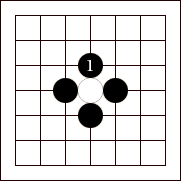In this case, the black play at “1” captures the white piece (and likely gains black an additional point for having surrounded territory at the end of the game)

More than one piece can be surrounded at a time, but not all configurations of stones can be surrounded.  Most notably, a configuration with two “eyes” (or empty holes)  cannot be captured, for example: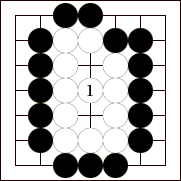Here, the white play at “1” creates two eyes.  There is no way for black to surround all of the white pieces since they would have to play in both empty holes, and as soon as black plays in one of the holes, the black piece is surrounded and immediately captured.

So, the main goal of Go (and the proof) revolves around creating “safe” structures that contain two eyes and using them to capture as much territory as possible.

Reduction: The paper by Lichtenstein and Sipser reduce from Planar Geography.  The idea is to have a set of safe territory for White, and another threatened set of stones large enough that if it can be made safe, White will win, but if it can be captured, Black will win.  Here’s the picture from the paper: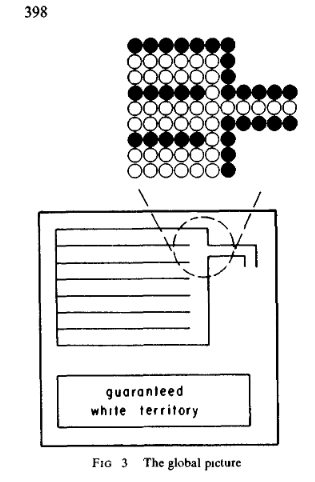Black pieces surround white all the way around, so the only escape would be for white to extend the “pipe” on the left around to the safe white spaces (or some other group of two eyes, which will keep the large group alive).  Then we encode the vertices and edges in the graph as sets of stones, where each “choice” of going through an edge is reflected by a choice of where stones are placed.

There are many types of subgraphs and corresponding board positions in the paper, here’s just one of them:

(Graph position)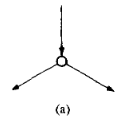(Board position)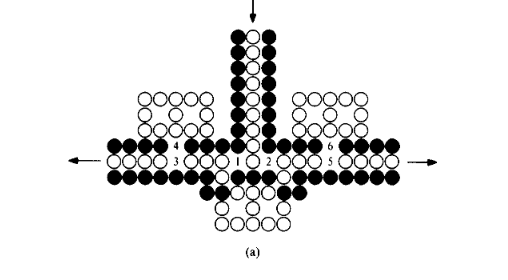Here’s the general structure of the arguments that show how the play “has” to go through this vertex.  Suppose we are coming from the top, and white wants to go left.

• If White doesn’t play at 1, 2, or 3 first, Black will play at 2.  White will now have to play at 1 to keep the middle vertical strip (which connects back to the big threatened set of pieces) alive.  But then Black plays at 3 and takes them all anyway.
• Even if White plays at 3, Black wins by playing at 1, then White moves to 2, then Black plays at 5
• If White does play at 1 or 2, (let’s say 1, because that will take us left), Black has to respond at the other point (so, 2 for us).  If they don’t, White plays at 2, and 3 black stones below the 1 and 2 are captured, and White will be able to connect to the two eye group below.
• After Black plays at 2, white needs to go to 3 to build a line of white stones coming in from the top, and going out to the left.  Black plays at 4 to stop white from connecting to the group of 2 eyes on the left.

As a result, the “edge” going through this vertex and coming out to the left has been chosen.

Difficulty: 7. I think this is easier to see than the NxN checkers reduction, but still takes a lot of cases and structures to realize.

## Planar Geography

In the process of reading up on the next problem in the appendix, I saw this nice reduction for a generalization of Geography that we used last week and will use next week.  So I thought it would make a nice addition to post it here.

The problem: Planar Geography.  This problem is not in the appendix (though the reference to this problem and the paper with this reduction appears in the notes to the “Generalized Geography” problem).

The description: Given an instance of the Generalized Geography problem, but where the graph G is planar, does Player 1 have a forced win?

Example: The example we had in the Generalized Geography problem is actually a planar graph: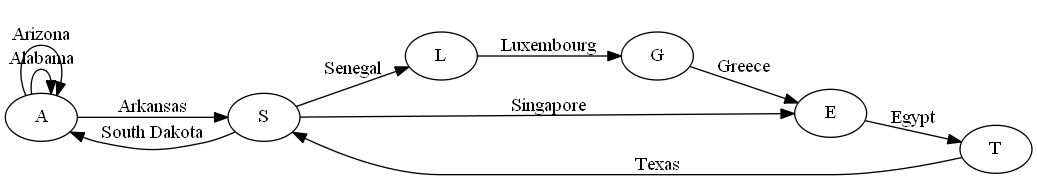Reduction: The paper by Lichtenstein and Sipser which has the reduction for next week’s NxN Go problem has this in the ramp-up.

What we’re doing to do is re-build the graph that was created in the Generalized Geography reduction.  That graph may not be planar. Any time the graph has two edges that cross: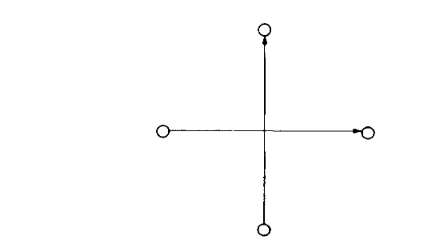We replace it with the following construction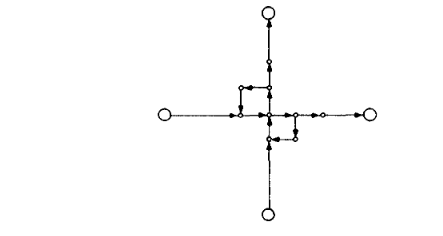(figures are on pages 395 and 396 of the paper).

The important things to realize are these:

1) If we use this on the graph generated from the original Geography Construction, it will never be the case that we will use both crossing edges in one solution.  This is an important point that isn’t really addressed in the paper.  The Lichtenstein and Sipser paper has a slightly different reduction for Generalized Geography where it’s easier to see.

2) Suppose it’s player 1’s turn, and they enter the intersection going up.  Then it will be their turn again once they hit the middle point.  The point of the extra loop structures (instead of just adding a vertex at the intersection point) is to create a situation where if player 1 decides to turn right, player 2 can turn down, and make player 1 lose.  This works in both directions.

Difficulty: I was hoping that this would be a good difficulty 4 or so problem in the middle of all of these really hard ones, but the whole “you’ll never use this intersection twice” problem makes the construction hard to see, and it’s a tough thing to think about why it matters, even if you told the student the rule.  Maybe this is a 5 if you give them that information.

## NxN Checkers

Back from my trip with a simple problem to explain, but a hard reduction to do.

The problem: NxN checkers.  This is problem GP10 in the appendix.

The description: Given a position on an NxN checkerboard, does black have a forced win?  It turns out the reduction will also work if we restrict the board to only having kings on the board (and so no “un-kinged” pieces)

Example: The “NxN” requirement is there since on a standard 8×8 checkerboard, there is a finite set of moves, and so theoretically you could solve the problem in O(1) time (for a really large constant factor, of course).  The starting configuration adds extra rows and columns of pieces to the board, still leaving two blank rows in between the two pieces.

So, let’s do an example on a 4×4 board.  The starting configuration is this:

 * – * – – – – – – – – – – O – O

(the dashes are empty spaces, * is Black, O is White)

Here is a configuration of pieces that will lead to a black win:

 – – – – – * – – O – – – – * – –

If it’s Black’s turn, they should move the piece in the second row up to either location on the first row (recall that all pieces are kings).  Then White’s only move is to go to the space Black just vacated, where it will be jumped, giving Black the win.

Reduction:

The paper by Fraenkel, Garey, Johnson, Schaefer, and Yesha contains a pretty detailed description of the reduction, which contains lots of complicated structures.  I’ll just give the general idea here.

The reduction is going to be from Geography, which is still NP-Complete even if the graph is bipartite and planar.  They create several structures to help them build their instance of the checkers game.

The first is what the call a phalanx– an open rectangle of (say) White kings that surround the (say) Black pieces.  The idea is that since there is no way for the Black pieces to jump anything in the rectangle, then White can “shrink” the phalanx towards Black, running them out of room to maneuver.  Here is a picture of a small phalanx on a 6×6 board:

 O O O O O O O X O O O O O O O O

..notice that whatever Black does, they will be captured on their next turn.  This remains true no matter how many Black pieces are trapped inside the phalanx, and no matter how much open space is inside the phalanx (White can use their moves to shrink it over time).

The key to the reduction is to build a set of interlocking “potential” phalanxes- situations where a Black king may be able to escape the phalanx.  If it can, Black can jump White’s pieces and win, but if it can’t, the phalanx will engulf Black and they will lose.  The geography instance is placed in the center of these potential phalanxes in such a way that a Black king can “escape” the Geography instance if and only if Black can win the geography game.  The reason why the Geography graph had to be planar was so that we could directly represent the vertices in the graph as positions on the checkerboard.  The reason why the Geography graph had to be bipartite was so that edges going from the first vertex set to the second could be all Black pieces, but the edges going from the second set to the first could be all White pieces.

The game starts with black at the “start vertex” for the geography problem, and jumping a line of White checkers: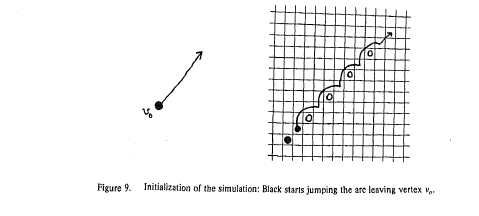When a vertex has more than one possible exit, that leads to more than one possible set of checkers to jump for the other player: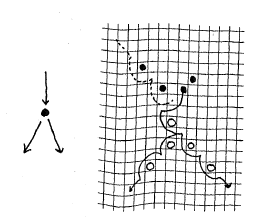(This is part of figure 10 from the paper.  Here, after White jumps down the chain of Black pieces, Black can choose the chain of White pieces to jump through.)

The construction takes advantage of the rule in checkers (which I was not aware of until I was in my twenties!) that if a player can make a jump, they must make a jump.  So as long as players can jump checkers along these chains (alternately, as long as they can follow edges in the geography graph), they will.  As soon as a player cannot make a jump they will be able to deal with the Black king that can either escape the phalanx structure (and win for Black) or trap it (and win for White).

This is the general idea of the reduction, there are a lot of details that I am glossing over.

Difficulty: 8.  This is a bit hard to see and very hard to come up with, and it’s very easy to get lost in the weeds of the details.  I do like the way that the “removal” of edges from the Geography problem is modeled by the actual removal of pieces from the checkerboard, though.

## Annihilation

I’m going to be out of town for a few weeks, so the next few posts might be delayed from my already slower schedule.

The problem: Annihilation.  This is problem GP9 in the appendix.

The description: G&J’s description is a little obscure, so we’ll go with the one in the paper by Fraenkel and Yesha that has the reduction.

Given a directed graph G=(V, E), and r subsets of E, E1 through Er.  The subsets may not be disjoint, but each edge of E is in at least one subset.

We’re also given r different types of tokens, placed on vertices of the graph.  Each token type corresponds to one of the r subsets of E.  A player moves by taking a token (of type i) and choosing an edge (u,v) in set Ei, where u is the current position of the token.  The token is moved to vertex v in the graph.  If 2 tokens ever meet on the same vertex, both are “annihilated” and removed from the game.  A player loses if they cannot make a move.  Does player 1 have a forced win?  (Though note that the Fraenkel and Yesha paper actually proves whether player 2 has a forced win)

Example: Here is a simple example that will hopefully be useful in the reduction that follows: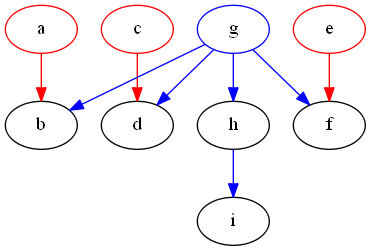In this graph, the red edges are in E1 and the blue edges are in E2.  The red vertices currently hold a type 1 token, and the blue vertex holds a type 2 token.

If player 1 makes any move except moving the token on g to the vertex h, player 2 will be able to move a red and a blue token onto the same space, annihilating both.  Then there will be just 2 moves left in the game (the 2 remaining red pieces moving along their edges), the first moved by player 1, the second moved by player 2, then player 1 will have no move and will lose.

If player 1 moves from g->h, then there are 4 more moves in the game (the h->i edge, and the three red edges).  Thus, player 1 will get the last move and will win.

Reduction: Fraenkel and Yesha use Minimum Cover.  I’ll note again here that he reduction will show that the Cover instance is true if and only if player two has a forced win.

So we’re given a collection of sets Si where i goes from 1 to m, and an integer K.  We’re going to build a directed acyclic bipartite graph R= (V, E):

• The graph has vertices xi and yi for i from 1 to K.
• The graph has one vertex for each set Si and one vertex for each element ei in the union of the sets.
• The graph also has two “special” vertices a and b.
• “Type 1” edges go from all xi to its corresponding yi, and from each yi to all Si vertices.
• “Type 2” edges go from a to all ei vertices, from all ei vertices to all set vertices that contain that element, between all e and x vertices, and from all x and S vertices to b.
• Type 1 pieces start on all x vertices.  There is 1 type 2 piece, and it’s on a.

Here is the example used in the paper for the covering problem {{e1,e2}, {e2,e3}, {e3,e4}, {e1,e3,e5}, {e6}} and K=4: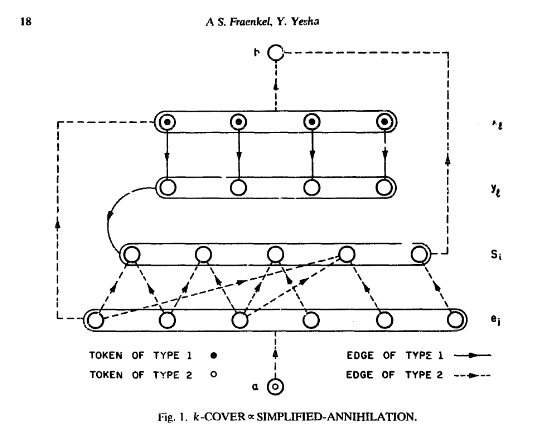An arrow going to a circled group of vertices represents a group of edges going to all vertices in the group.

Notice that without annihilation, the path a type 1 piece takes is from some x vertex to its y vertex, and from there to some S vertex (2 moves), and the path of the type 2 piece is 3 moves (either a->some e-> some x ->b or a->some e->some S ->b), so there is an odd number of moves, and thus player 1 wins if no annihilations happen.  Player 2 wins if a type 1 and type 2 piece collide someplace (on an x or S vertex).

This is because if 2 pieces of different types collide, we remove an odd number of moves from the game:

• If they collide on an x vertex, we remove the 2 moves the type 1 piece can make, and the move the type 2 piece could make from x->b
• If they collide on an S vertex, we remove the one move the type 2 piece makes from S->b

On player 1’s move, they will have to move all pieces off of the x vertices before moving the type 2 token off of the a vertex.  Otherwise, after player 1 moves a->e, player 2 can move e->x (to some x that hasn’t left its starting space yet).

So,  player 1 starts by moving some xi->yi.  Player 2 will move the piece from yi to the next set in the cover.  Recall that there are k different x and y vertices.  So what will happen is that the k vertices that comprise the cover will have tokens on them.

Once all of the type 1 pieces are some S vertex, player 1 will have to move the type 2 piece from a to some e vertex.  If there is a cover, no matter what e vertex player 1 chooses, player 2 will be able to move the token to an S vertex that contains that e element.  If there is no cover, player 1 will be able to choose an e vertex that has no e->S (or e->x) move that causes an annihilation, and player 1 will win.

Difficulty: 7.  This is a very cool reduction, and you can see from the picture how it works.  It’s fun to see how all of the edges and sets work out.

## Alternating Maximum Weighted Matching

This is a “private communication” problem I haven’t been able to solve or find an actual reduction for.  I think I have the start of a reduction, though, but I haven’t put in the time to work out the details.  Hopefully, I’m on the right track.

The problem: Alternating Maximum Weighted Matching.  This is problem GP8 in the appendix.

The description: Given a weighted graph G=(V,E), with positive weights, and a positive bound B, each player chooses an edge from E.  No two chosen edges can share an endpoint.  Player 1 wins if the weights of the edges chosen ever exceed B.  Does player 1 have a forced win?

Example: Here’s a graph: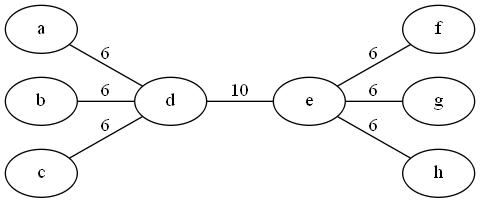If B=11, and it’s player 1’s turn, then they can’t remove the highest-cost edge (d,e) because every edge is incident on d or e, so player 2 would have no moves, and the game would end with cost 10.

If player 1 removes one of the 6-cost edges (let’s say (a,d)) we’re left with: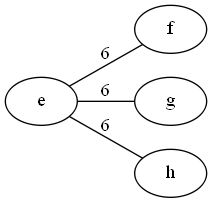..and so player 2 will have to take one of the remaining 6-cost edges, bringing the total cost of edges removed to 12.

Reduction (sort of): So, like I said at the top, the reference in G&J is to a “private communication” by Dobkin and Ladner.  I couldn’t find the actual result published anywhere.  I actually emailed both Dobkin and Ladner to ask if they remembered what the reduction was, but their response (reasonably) was “It’s been 40 years, I have no idea”.

But, I thought about it for a little while, at least, and came up with what I thought are the beginnings of an idea.  I didn’t have the time (or, perhaps, the ability) to get all of the details right, but this feels like a start, at least:

We’re going to reduce from One in Three 3SAT.  Each variable will be represented as a pair of vertices xi and xia, connected by a weight 1 edge.  The negation of the variable (~xi and ~xia) will also be vertices and also connected by a weight 1 edge.  The vertices xi and ~xi will be connected by a “large” edge of weight 10.

From each clause, we build up a component of a graph that looks like this: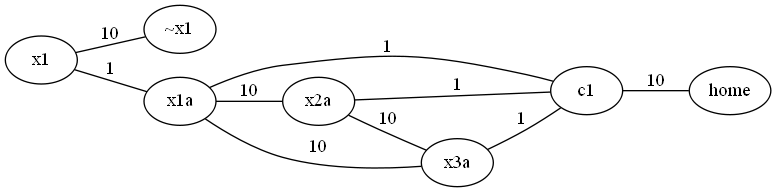The weights of 10 and 1 might not be right, think of them as “large” and “small” weights.  Each of the xi variables corresponds to the actual variables in the formula, we only include the variables that correspond to the clause we’re looking at.

(One thing I did wrong was to assume that player 1 loses if the edge cost goes over B.  So in what follows, player 1 is trying to keep the score low.  We can fix this by making it player 2’s turn and swapping the roles)

The idea is that player 1 will choose either the x1-x1a edge or the ~x1-~x1a edge to “fix” the value of the first variable.  If the variable shows up in the clause (for example, they chose the x1-x1a edge in the diagram above), this will eliminate the edges (x1a,c1), (x1a, x2a), and (x1a, x3a) from being able to be chosen.

Then player 2 will want to choose an “expensive” edge.  He’ll choose the edge (x2a,x3a)

Then we’ll move on to the next variable.  It’s again player 1’s turn to decide on a setting of x2.

The idea is that each clause will have it’s “ci” vertex connect to that central “home” vertex by an expensive edge, so if after all of the variables have been given their values, there still is an edge to the home vertex, it will be chosen, and that amount will be the amount that sends the total cost over the bound.  (So right now, I’m thinking of the bound being something like 11*N +1 for a problem with N variables, at least until the extra things below get added).

What still needs to be done is the detail work (and probably extra edges and vertices) to ensure that players have to choose the edges in the order I specify (i.e., not doing so loses a player the game immediately).  It’s entirely possible that doing so will make this whole construction wrong.  But I like the idea behind it, at least

Difficulty: N/A.  I don’t want to call this a 10, even though it stumped me.  I think if my idea is right, it’s not that hard.

## Alternating Hitting Set

I think this might be the last of the problems we do from the Schaefer paper we’ve used so much recently.

The problem: Alternating Hitting Set.  This is problem GP7 in the appendix.

The description: Given some universe set B, and a collection C of subsets of B.  Players take turns choosing an element from B.  Once enough elements are chosen to make all sets in C have at least one element chosen, the player who made that move loses.  Does player 1 have a forced win?

Example: Let B= {1,2,3}, and C = {{1,2,3}, {2,3}, {1,3}, {1,2}}.  Player 1 can win by choosing 1, which hits all sets in C except {2,3}.  Since player 2 has to pick either a 2 or 3, they will hit that set and lose.

Reduction: Schaefer remarks that this is just a special case of Variable Partition Truth Assignment. with sets instead of variables.  Here’s how that goes:

Suppose we have an instance of Variable Partition Truth Assignment, so a CNF formula with all positive variables.  We just create a set B with one element per variable, and the clauses become the sets in C.  Then picking an element of B is the same as setting a variable to true.  Hitting a set in C is the same as making the clause true.

Difficulty: 3.  Maybe this problem is close enough to Variable Partition Truth Assignment that it didn’t need its own article.  On the other hand, it’s nice to see an easy reduction for once.